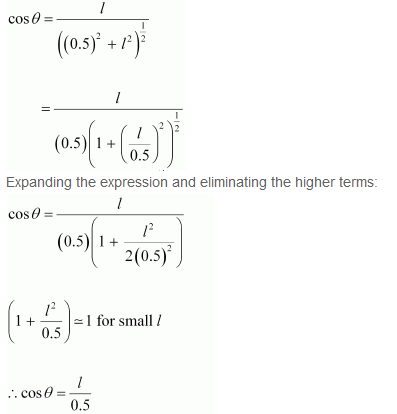## Pages

### Mechanical Properties of solids NCERT Solutions Class 11 Physics - Solved Exercise Question 9.19

Question 9.19:
A mild steel wire of length 1.0 m and cross-sectional area 0.50 × 10–2 cm2 is stretched, well within its elastic limit, horizontally between two pillars. A mass of 100 g is suspended from the mid-point of the wire. Calculate the depression at the midpoint.
Solution:Length of the steel wire = 1.0 m
Area of cross-section, A = 0.50 × 10–2 cm2 = 0.50 × 10–6 m2
A mass 100 g is suspended from its midpoint.
m = 100 g = 0.1 kg
Hence, the wire dips, as shown in the given figure.Original length = XZ
Depression = l
The length after mass m, is attached to the wire = XO + OZ
Increase in the length of the wire:
Δl = (XO + OZ) – XZ

Where,
Expanding and neglecting higer terms, we get:
∆l = l2 / 0.5
Strain = Increase in length / Original length
Let T be the tension in the wire.
∴ mg = 2T cosθ

Using the figure, it can be written as:Hence, the depression at the midpoint is 0.0106 m.